Courses

# Time Value of Money (Part - 2) CA CPT Notes | EduRev

## CA CPT : Time Value of Money (Part - 2) CA CPT Notes | EduRev

The document Time Value of Money (Part - 2) CA CPT Notes | EduRev is a part of the CA CPT Course Business Mathematics and Logical Reasoning & Statistics.
All you need of CA CPT at this link: CA CPT

4.5 EFFECTIVE RATE OF INTEREST

If interest is compounded more than once a year the effective interest rate for a year exceeds the per annum interest rate. Suppose you invest Rs.10000 for a year at the rate of 6% per annum compounded semi annually. Effective interest rate for a year will be more than 6% per annum since interest is being compounded more than once a year. For computing effective rate of interest first we have to compute the interest. Let us compute the interest.

Interest for first six months = Rs. 10000 × 6/100 × 6/12
= Rs. 300
Principal for calculation of interest for next six months
= Principal for period one + interest for period one
= Rs. (10000 + 300)
= Rs. 10300
Interest for next six months = Rs. 10300 × 6/100 × 6/12 = Rs. 309
Total interest earned during the current year
= interest for first six months + interest for next six months
= Rs.(300 + 309) = Rs. 609
Interest of Rs. 609 can also be computed directly from the formula of compound interest.
We can compute effective rate of interest by following formula
I = PEt
Where I = amount of interest
E = effective rate of interest in decimal
t = time period
P = principal amount
Putting the values we have
609 = 10000 × E × 1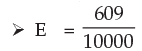= 0.0609 or
= 6.09%

Thus if we compound the interest more than once a year effective interest rate for the year will be more than actual interest rate per annum. But if interest is compounded annually effective interest rate for the year will be equal to actual interest rate per annum.
So effective interest rate can be defined as the equivalent annual rate of interest compounded annually if interest is compounded more than once a year.

 The effective interest rate can be computed directly by following formula:E = (1 + i)n – 1Where E is the effective interest ratei = actual interest rate in decimaln = number of conversion period

Example 22: Rs. 5000 is invested in a Term Deposit Scheme that fetches interest 6% per annum compounded quarterly. What will be the interest after one year? What is effective rate of interest?

Solution: We know that I= P [(1+i)n−1]
Here P = Rs. 5000
i = 6% p.a. = 0.06 p.a. or 0.015 per quarter
n= 4
and I = amount of compound interest
putting the values we have
I = Rs. 5000 [(1+0.015)4−1]
= Rs. 5000 × 0.06136355
= Rs. 306.82
For effective rate of interest using I = PEt we find
306.82 = 5000 × E × 1.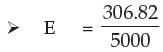= 0.0613 or 6.13%
Note: We may arrive at the same result by using
E = (1+i)n – 1
E = (1 + 0.015)4 - 1
= 1.0613 - 1
= .0613 or 6.13%

We may also note that effective rate of interest is not related to the amount of principal. It is related to the interest rate and frequency of compounding the interest

Example 23: Find the compound interest and effective rate of interest if an amount of Rs.20000 is deposited in a bank for one year at the rate of 8% per annum compounded semi annually.

Solution: We know that I= P [(1+i)n−1] here P = Rs. 20000
i = 8% p.a.     = 8/2 % semi annually = 0.04
n= 2
I = Rs. 20000 [(1+0.04)2−1]
= Rs. 20000 x 0.0816
= Rs. 1632

Effective rate of interest:

We know that

I = PEt
1632 = 20000 × E × 1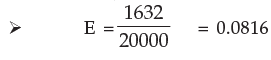= 8.16%
Effective rate of interest can also be computed by following formula
E = (1 + i)n -1
= (1 + 0.04)2 -1
= 0.0816 Or  8.16%

Example 24: Which is a better investment 3% per year compounded monthly or 3.2% per year simple interest? Given that (1+0.0025)12 =1.0304.

Solution: i = 3/12 = 0.25% = 0.0025
n= 12
E = (1 + i)n - 1
= (1 + 0.0025)12 - 1
= 1.0304 – 1 = 0.0304
= 3.04%
Effective rate of interest (E) being less than 3.2%, the simple interest 3.2% per year is the better investment.

4.6 ANNUITY

In many cases you must have noted that your parents have to pay an equal amount of money regularly like every month or every year. For example payment of life insurance premium, rent of your house (if you stay in a rented house), payment of housing loan, vehicle loan etc. In all these cases they pay a constant amount of money regularly. Time period between two consecutive payments may be one month, one quarter or one year.
Sometimes some people received a fixed amount of money regularly like pension rent of house etc. In all these cases annuity comes into the picture. When we pay (or receive) a fixed amount of money periodically over a specified time period we create an annuity.
Thus annuity can be defined as a sequence of periodic payments (or receipts) regularly over a specified period of time.
There is a special kind of annuity also that is called Perpetuity. It is one where the receipt or payment takes place forever. Since the payment is forever we cannot compute a future value of perpetuity. However we can compute the present value of the perpetuity. We will discuss later about future value and present value of annuity.
To be called annuity a series of payments (or receipts) must have following features:
(1) Amount paid (or received) must be constant over the period of annuity and
(2) Time interval between two consecutive payments (or receipts) must be the same.
Consider following tables. Can payments/receipts shown in the table for five years be called annuity?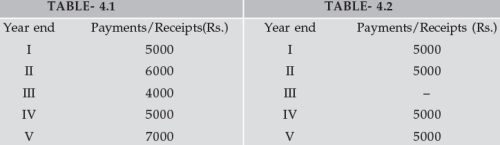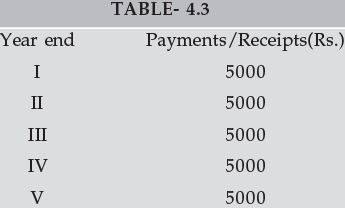Payments/Receipts shown in table 4.1 cannot be called annuity. Payments/Receipts though have been made at regular intervals but amount paid are not constant over the period of five years.
Payments/receipts shown in table 4.2 cannot also be called annuity. Though amounts paid/ received are same in every year but time interval between different payments/receipts is not equal. You may note that time interval between second and third payment/receipt is two year and time interval between other consecutive payments/receipts (first and second third and fourth and fourth and fifth) is only one year. You may also note that for first two year the payments/receipts can be called annuity.
Now consider table 4.3. You may note that all payments/receipts over the period of 5 years are constant and time interval between two consecutive payments/receipts is also same i.e. one year. Therefore payments/receipts as shown in table-4.3 can be called annuity.

4.6.1 Annuity regular and Annuity due/immediate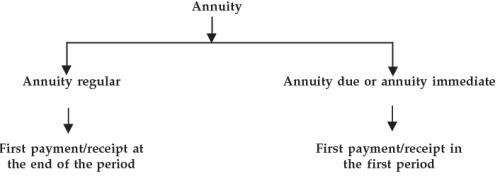Annuity may be of two types:

(1) Annuity regular: In annuity regular first payment/receipt takes place at the end of first period. Consider following table: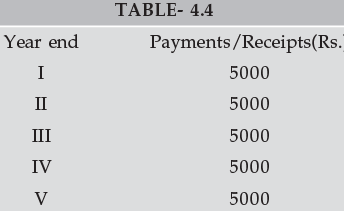We can see that first payment/receipts takes place at the end of first year therefore it is an annuity regular.

(2) Annuity Due or Annuity Immediate: When the first receipt or payment is made today ( at the beginning of the annuity) it is called annuity due or annuity immediate. Consider following table: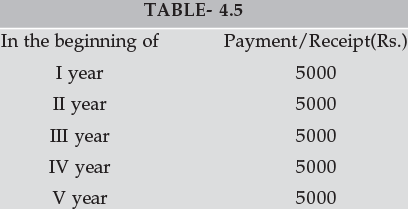We can see that first receipt or payment is made in the beginning of the first year. This type of annuity is called annuity due or annuity immediate.

4.7 FUTURE VALUE

Future value is the cash value of an investment at some time in the future. It is tomorrow’s value of today’s money compounded at the rate of interest. Suppose you invest Rs.1000 in a fixed deposit that pays you 7% per annum as interest. At the end of first year you will have Rs.1070. This consist of the original principal of Rs.1000 and the interest earned of Rs.70.
Rs.1070 is the future value of Rs.1000 invested for one year at 7%. We can say that Rs.1000 today is worth Rs.1070 in one year’s time if the interest rate is 7%.
Now suppose you invested Rs.1000 for two years. How much would you have at the end of the second year. You had Rs.1070 at the end of the first year. If you reinvest it you end up having Rs.1070(1+0.07)=Rs.1144.90 at the end of the second year. Thus Rs.1144.90 is the future value of Rs.1000 invested for two years at 7%. We can compute the future value of a single cash flow by applying the formula of compound interest.

We know that
An = P(1+i)n
Where A = Accumulated amount
n = number of conversion period
i = rate of interest per conversion period in decimal
P = principal
Future value of a single cash flow can be computed by above formula. Replace A by future value (F) and P by single cash flow (C.F.) therefore
F = C.F. (1 + i)n

Example 25: You invest Rs. 3000 in a two year investment that pays you 12% per annum.
Calculate the future value of the investment.

Solution: We know
F = C.F. (1 + i)n
where F = Future value C.F. = Cash flow = Rs.3000
i = rate of interest = 0.12
n = time period = 2
F = Rs. 3000(1+0.12)2
= Rs. 3000×1.2544
= Rs. 3763.20

4.7.1 Future value of an annuity regular :
Now we can discuss how do we calculate future value of an annuity.
Suppose a constant sum of Re. 1 is deposited in a savings account at the end of each year for four years at 6% interest. This implies that Re.1 deposited at the end of the first year will grow for three years, Re. 1 at the end of second year for 2 years, Re.1 at the end of the third year for one year and Re.1 at the end of the fourth year will not yield any interest. Using the concept of compound interest we can compute the future value of annuity. The compound value (compound amount) of Re.1 deposited in the first year will be
A3 = Rs. 1 (1 + 0.06)= Rs. 1.191

The compound value of Re.1 deposited in the second year will be
A2 = Rs. 1 (1 + 0.06)2
= Rs. 1.124

The compound value of Re.1 deposited in the third year will be
A1 = Rs. 1 (1 + 0.06)1
= Rs. 1.06
and the compound value of Re. 1 deposited at the end of fourth year will remain Re. 1.
The aggregate compound value of Re. 1 deposited at the end of each year for four years would be:
Rs. (1.191 + 1.124 + 1.060 + 1.00) = Rs. 4.375
This is the compound value of an annuity of Re.1 for four years at 6% rate of interest.
The above computation is summarized in the following table: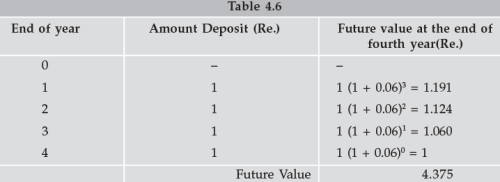The computation shown in the table can be expressed as follows:
A (4, i) = A (1 + i)+ A (1 + i) + A(1 + i)2 + A( 1 + i)3
i.e. A (4, i) = A[1+ (1+i) +(1+i)2 +(1 + i)3]

In above equation A is annuity, A (4, i) is future value at the end of year four, i is the rate of interest shown in decimal.
We can extend above equation for n periods and rewrite as follows:
A (n, i) = A (1 + i)0 + A (1 + i)1 + .......................... +A (1 + i)n-2 + A (1 + i)n-1
Here A = Re.1
Therefore A (n, i) = 1 (1 + i)0 + 1 (1 + i)1 + .......................... +1 (1 + i)n-2 + 1 (1 + i)n-1
= 1 + (1 + i)1 + .......................... + (1 + i)n-2 + (1 + i)n-1

[a geometric series with first term 1 and common ratio (1+ i)]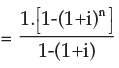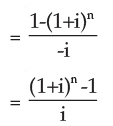If A be the periodic payments, the future value A(n, i) of the annuity is given by
A(n, i) = A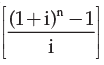Example 26: Find the future value of an annuity of Rs.500 made annually for 7 years at interest rate of 14% compounded annually. Given that (1.14)7 = 2.5023.

Solution: Here annual payment A = Rs.500
n= 7
i = 14% = 0.14
Future value of the annuity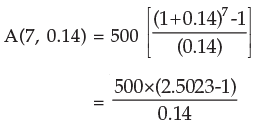Example 27: Rs. 200 is invested at the end of each month in an account paying interest 6% per year compounded monthly. What is the future value of this annuity after 10th payment? Given that (1.005)10 = 1.0511

Solution: Here A = Rs.200
n = 10
i = 6% per annum = 6/12 % per month = 0.005
Future value of annuity after 10 months is given by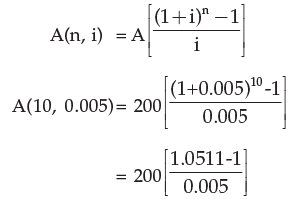= 200×10.22
= Rs. 2044

4.7.2 Future value of Annuity due or Annuity Immediate: As we know that in Annuity due or Annuity immediate first receipt or payment is made today. Annuity regular assumes that the first receipt or the first payment is made at the end of first period. The relationship between the value of an annuity due and an ordinary annuity in case of future value is:
Future value of an Annuity due/Annuity immediate = Future value of annuity regular x (1+i) where i is the interest rate in decimal.
Calculating the future value of the annuity due involves two steps.
Step-1 Calculate the future value as though it is an ordinary annuity.
Step-2 Multiply the result by (1+ i)

Example 28: Z invests Rs. 10000 every year starting from today for next 10 years. Suppose interest rate is 8% per annum compounded annually. Calculate future value of the annuity.
Given that (1 + 0.08)10 = 2.15892500.

Solution: Step-1: Calculate future value as though it is an ordinary annuity.
Future value of the annuity as if it is an ordinary annuity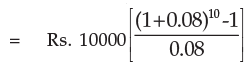= Rs. 10000 × 14.4865625
= Rs. 144865.625

Step-2: Multiply the result by (1 + i)
= Rs. 144865.625 × (1+0.08)
= Rs. 156454.875

4.8 PRESENT VALUE

We have read that future value is tomorrow’s value of today’s money compounded at the interest rate. We can say present value is today’s value of tomorrow’s money discounted at the interest rate. Future value and present value are related to each other in fact they are the reciprocal of each other. Let’s go back to our fixed deposit example. You invested Rs. 1000 at 7% and get Rs. 1070 at the end of the year. If Rs. 1070 is the future value of today’s Rs. 1000 at 7% then Rs. 1000 is present value of tomorrow’s Rs. 1070 at 7%. We have also seen that if we invest Rs. 1000 for two years at 7% per annum we will get Rs. 1144.90 after two years. It means Rs. 1144.90 is the future value of toady’s Rs. 1000 at 7% and Rs. 1000 is the present value of Rs. 1144.90 where time period is two years and rate of interest is 7% per annum. We can get the present value of a cash flow (inflow or outflow) by applying compound interest formula.

 The present value P of the amount An due at the end of n interest period at the rate of i per interest period may be obtained by solving for P the equation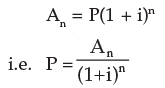Computation of P may be simple if we make use of either the calculator or the present value table showing values of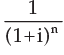for various time periods/per annum interest rates. For positive i the factoris always less than 1 indicating thereby future amount has smaller present value.

Example 29: What is the present value of Re.1 to be received after two years compounded annually at 10% ?

Solution: Here A= Re.1
i = 10% = 0.1
n= 2
Required present value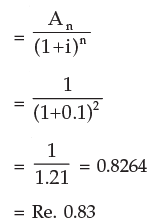Thus Re. 0.83 shall grow to Re. 1 after 2 years at 10% compounded annually.

Example 30: Find the present value of Rs. 10000 to be required after 5 years if the interest rate be 9%. Given that (1.09)5=1.5386.

Solution: Here i = 0.09
n =5
An= 10000

Required present value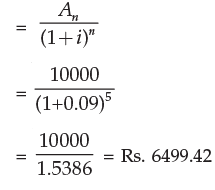4.8.1 Present value of an Annuity regular: We have seen how compound interest technique can be used for computing the future value of an Annuity. We will now see how we compute present value of an annuity. We take an example. Suppose your mom promise you to give you Rs.1000 on every 31st December for the next five years. Suppose today is 1st January. How much money will you have after five years from now if you invest this gift of the next five years at 10%? For getting answer we will have to compute future value of this annuity.

But you don’t want Rs. 1000 to be given to you each year. You instead want a lump sum figure today. Will you get Rs. 5000. The answer is no. The amount that she will give you today will be less than Rs. 5000. For getting the answer we will have to compute the present value of this annuity. For getting present value of this annuity we will compute the present value of these amounts and then aggregate them. Consider following table: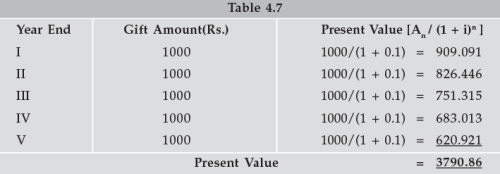Thus the present value of annuity of Rs. 1000 for 5 years at 10% is Rs. 3790.79
It means if you want lump sum payment today instead of Rs.1000 every year you will get Rs. 3790.79.
The above computation can be written in formula form as below.
The present value (V) of an annuity (A) is the sum of the present values of the payments.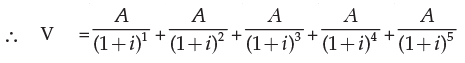We can extend above equation for n periods and rewrite as follows: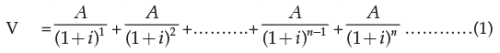multiplying throughout by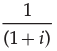we get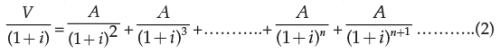subtracting (2) from (1) we get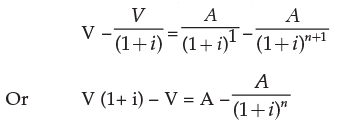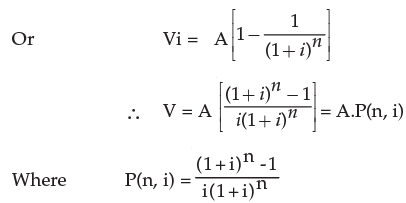Consequently A =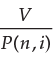which is useful in problems of amortization.

A loan with fixed rate of interest is said to be amortized if entire principal and interest are paid over equal periods of time by way of sequence of equal payment.

A =can be used to compute the amount of annuity if we have present value (V), n the number of time period and the rate of interest in decimal.Suppose your dad purchases a car for Rs. 550000. He gets a loan of Rs. 500000 at 15% p.a. from a Bank and balance 50000 he pays at the time of purchase. Your dad has to pay whole amount of loan in 12 equal monthly instalments with interest starting from the end of first month.Now we have to calculate how much money has to be paid at the end of every month. We can compute equal instalment by following formula

A =Here V = Rs. 500000
n = 12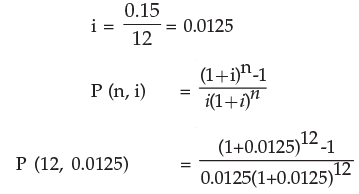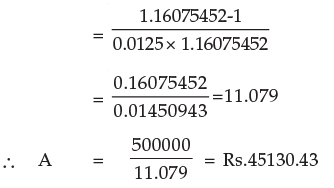Therefore your dad will have to pay 12 monthly instalments of Rs. 45130.43.

Example 31: S borrows Rs. 500000 to buy a house. If he pays equal instalments for 20 years and 10% interest on outstanding balance what will be the equal annual instalment?

Solution: We know

A =Here V = Rs.500000
n= 20
i = 10% p.a.= 0.10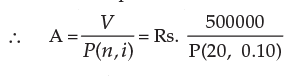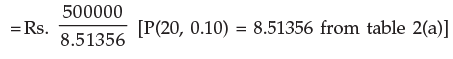= Rs. 58729.84

Example 32: Rs. 5000 is paid every year for ten years to pay off a loan. What is the loan amount if interest rate be 14% per annum compounded annually?

Solution: V = A.P.(n, i)
Here A = Rs. 5000
n = 10

i = 0.14
V = 5000 × P(10, 0.14)
= 5000 × 5.21611 = Rs. 26080.55
Therefore the loan amount is Rs. 26080.55 Note: Value of P(10, 0.14) can be seen from table 2(a) or it can be computed by formula derived in preceding paragraph.

Example 33: Y bought a TV costing Rs. 13000 by making a down payment of Rs. 3000 and agreeing to make equal annual payment for four years. How much would be each payment if the interest on unpaid amount be 14% compounded annually?

Solution: In the present case we have present value of the annuity i.e. Rs. 10000 (13000-3000) and we have to calculate equal annual payment over the period of four years.
We know that
V = A.P (n, i)
Here n = 4 and  i = 0.14

A =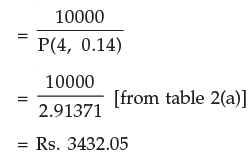Therefore each payment would be Rs. 3432.05

4.8.2 Present value of annuity due or annuity immediate:
Present value of annuity due/ immediate for n years is the same as an annuity regular for (n-1) years plus an initial receipt or payment in beginning of the period. Calculating the present value of annuity due involves two steps.
Step 1: Compute the present value of annuity as if it were a annuity regular for one period short.
Step 2: Add initial cash payment/receipt to the step 1 value.

Example 34: Suppose your mom decides to gift you Rs. 10000 every year starting from today for the next five years. You deposit this amount in a bank as and when you receive and get 10% per annum interest rate compounded annually. What is the present value of this annuity?

Solution: It is an annuity immediate. For calculating value of the annuity immediate following steps will be followed:

Step 1: Present value of the annuity as if it were a regular annuity for one year less i.e. for four years
= Rs. 10000 × P(4, 0.10)
= Rs. 10000 × 3.16987
= Rs. 31698.70

Step 2 : Add initial cash deposit to the step 1 value
Rs. (31698.70+10000) = Rs. 41698.70

4.9 SINKING FUND

It is the fund credited for a specified purpose by way of sequence of periodic payments over a time period at a specified interest rate. Interest is compounded at the end of every period. Size of the sinking fund deposit is computed from A = P.A(n, i) where A is the amount to be saved, P the periodic payment, n the payment period.

Example 35: How much amount is required to be invested every year so as to accumulate Rs. 300000 at the end of 10 years if interest is compounded annually at 10%?

Solution: Here A = 300000
n= 10
i = 0.1
Since A = P.A (n, i)
300000 = P.A.(10, 0.1)
= P × 15.9374248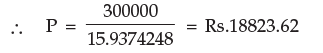This value can also be calculated by the formula of future value of annuity regular.
We know that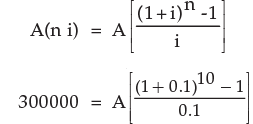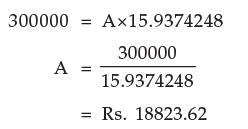4.10 APPLICATIONS

4.10.1 Leasing: Leasing is a financial arrangement under which the owner of the asset (lessor) allows the user of the asset (lessee) to use the asset for a defined period of time(lease period) for a consideration (lease rental) payable over a given period of time. This is a kind of taking an asset on rent. How can we decide whether a lease agreement is favourable to lessor or lessee, it can be seen by following example.

Example 36: ABC Ltd. wants to lease out an asset costing Rs. 360000 for a five year period. It has fixed a rental of Rs. 105000 per annum payable annually starting from the end of first year. Suppose rate of interest is 14% per annum compounded annually on which money can be invested by the company. Is this agreement favourable to the company?

Solution: First we have to compute the present value of the annuity of Rs. 105000 for five years at the interest rate of 14% p.a. compounded annually.
The present value V of the annuity is given by
V = A.P (n, i)
= 105000 × P(5, 0.14)
= 105000 × 3.43308 = Rs. 360473.40
which is greater than the initial cost of the asset and consequently leasing is favourable to the lessor.

Example 37: A company is considering proposal of purchasing a machine either by making full payment of Rs.4000 or by leasing it for four years at an annual rate of Rs.1250. Which course of action is preferable if the company can borrow money at 14% compounded annually?

Solution: The present value V of annuity is given by V = A.P (n, i)
= 1250 × P (4, 0.14)
= 1250 × 2.91371 = Rs.3642.11
which is less than the purchase price and consequently leasing is preferable.

4.10.2 Capital Expenditure (investment decision): Capital expenditure means purchasing an asset (which results in outflows of money) today in anticipation of benefits (cash inflow) which would flow across the life of the investment. For taking investment decision we compare the present value of cash outflow and present value of cash inflows. If present value of cash inflows is greater than present value of cash outflows decision should be in the favour of investment. Let us see how do we take capital expenditure (investment) decision.

Example 38: A machine can be purchased for Rs.50000. Machine will contribute Rs.12000 per year for the next five years. Assume borrowing cost is 10% per annum compounded annually.
Determine whether machine should be purchased or not.

Solution: The present value of annual contribution
V = A.P(n, i)

= 12000 × P(5, 0.10)
= 12000 × 3.79079
= Rs. 45489.48

which is less than the initial cost of the machine. Therefore machine must not be purchased.

Example 39: A machine with useful life of seven years costs Rs. 10000 while another machine with useful life of five years costs Rs. 8000. The first machine saves labour expenses of Rs. 1900 annually and the second one saves labour expenses of Rs. 2200 annually. Determine the preferred course of action. Assume cost of borrowing as 10% compounded per annum.

Solution: The present value of annual cost savings for the first machine
= Rs. 1900 × P (7, 0.10)
= Rs. 1900 × 4.86842
= Rs. 9249.99
= Rs. 9250

Cost of machine being Rs. 10000 it costs more by Rs. 750 than it saves in terms of labour cost.
The present value of annual cost savings of the second machine
= Rs. 2200 × P(5, 0.10)
= Rs. 2200 × 3.79079
= Rs. 8339.74

Cost of the second machine being Rs. 8000 effective savings in labour cost is Rs. 339.74. Hence the second machine is preferable.

4.10.3 Valuation of Bond: A bond is a debt security in which the issuer owes the holder a debt and is obliged to repay the principal and interest. Bonds are generally issued for a fixed term longer than one year.

Example 40: An investor intends purchasing a three year Rs. 1000 par value bond having nominal interest rate of 10%. At what price the bond may be purchased now if it matures at par and the investor requires a rate of return of 14%?

Solution: Present value of the bond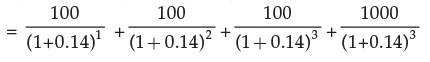= 100 × 0.87719 + 100 × 0.769467 + 100 × 0.674 972 + 1000 × 0.674972
= 87.719+ 76.947+ 67.497+ 674.972
= 907.125
Thus the purchase value of the bond is Rs.907.125

Offer running on EduRev: Apply code STAYHOME200 to get INR 200 off on our premium plan EduRev Infinity!

69 docs|76 tests

,

,

,

,

,

,

,

,

,

,

,

,

,

,

,

,

,

,

,

,

,

;